# Dimension ParametersThe dimension parameters are located in the Dimension node of the standard file. They deal with the appearance of annotation and dimension elements.

These parameters are global, which means that changing their value will have an impact on all elements in the drawing.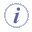This section lists all the parameters which were contained in CATDrwStandard files up to V5 R9.

### Dimension and Annotation Parameters

 Parameter Value Description International standard [ISO/ANSI/JIS] Each user-defined standard is based on one of 3 international standards: ISO, ANSI, JIS. This sets some basic parameters. Dimension Line: Extension on radius dimensions (value inside circle), Reach center [Yes/No] Yes = till center No = till value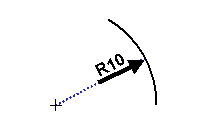Dimension Line: Extension on radius dimensions (value outside circle), Reach center [Yes/No] Yes = till center No = constant overrun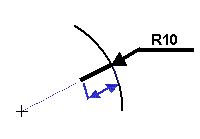Dimension Line: Extension on radius dimensions (value outside circle), Overrun length (mm) Dimension Line: Extension on one-symbol diameter dimensions (value inside circle), Reach center [Yes/No] Yes = till center No = till value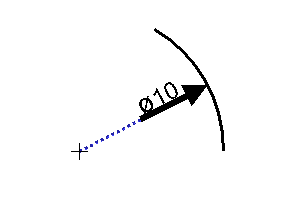Dimension Line: Extension on one-symbol diameter dimensions (value inside circle), Overrun length (mm) Dimension Line: Extension on one-symbol diameter dimensions (value outside circle), Reach center [Yes/No] Yes = till center No = constant overrun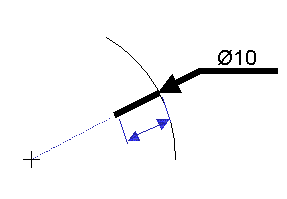Dimension Line: Extension on one-symbol diameter dimensions (value outside circle), Overrun length (mm) Dimension Line: Display and extent (for non-flipped symbols), Overrun length (mm)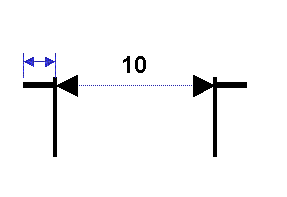Dimension Line: Display and extent (for non-flipped symbols), Show [Yes/No] Yes = displayed No = not displayed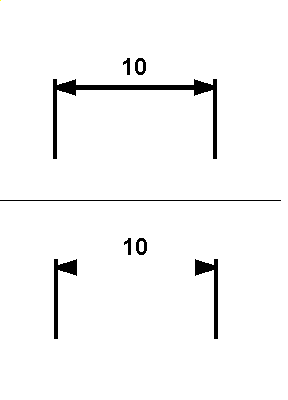Dimension Line: Display and extent (for flipped symbols), Overrun length (mm)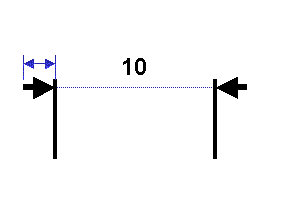Dimension Line: Display and extent (for flipped symbols), Show [Yes/No] Yes = displayed No = not displayed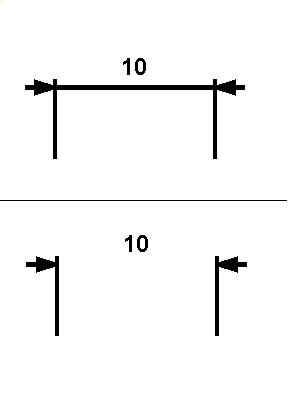Dimension Line: Length for one-symbol dimensions (distance and angle), Underline [2/1] 2 = Length relative to value 1 = Constant length The dimension line may either have a given length, or automatically adjust to reach the dimension value. Dimension Line: Length for one-symbol dimensions (distance and angle), Constant length (mm) if Dimension Line: Length for one-symbol dimensions (distance and angle), Underline = 1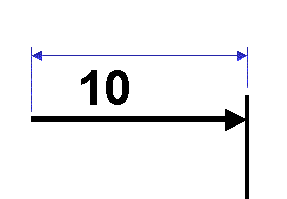Dimension Line: Gap around unframed value (mm)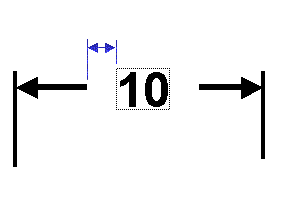Dimension Line: Gap around framed value (mm)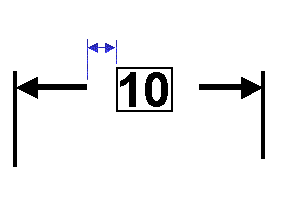DIMTYPos - Deprecated Dimension Value: Vertical justification [ 2 / 3 ] 2 = center 3 = bottom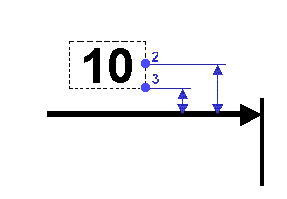Dimension tolerance: Multi-tolerance with associative numerical value [No/Yes] No = not associative Yes = associative Specifies whether the numerical definition of a multi-tolerance is associative to the dimension value (in which case it is automatically updated when the dimension value is changed).

### Dimension and Annotation Leader SymbolsNote that dimension and annotation leader symbols do not apply to arrows. If you want to modify symbol parameters for arrows, refer to the Dress-up Parameters > Symbols section.
 Parameter Value Description Dimension and Annotation Leader Symbols: Open arrow size (simple and double arrows) (mm)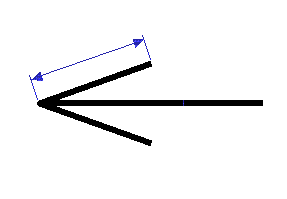Dimension and Annotation Leader Symbols: Open arrow angle (simple and double arrows) (degrees)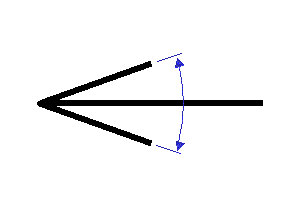Dimension and Annotation Leader Symbols: Outlined arrow size (mm)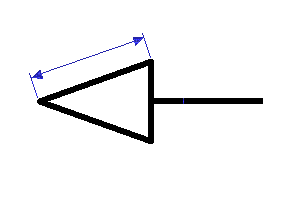Dimension and Annotation Leader Symbols: Outlined arrow angle (degrees)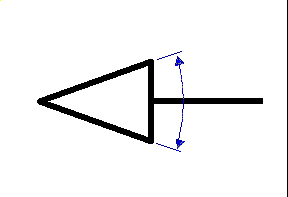Dimension and Annotation Leader Symbols: Filled arrow size (simple and double arrows) (mm)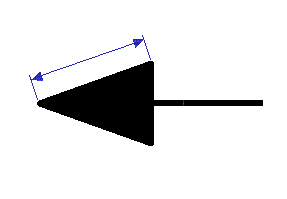Dimension and Annotation Leader Symbols: Filled arrow angle (simple and double arrows) (degrees)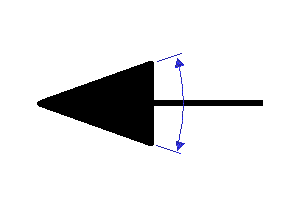Dimension and Annotation Leader Symbols: Transparent arrow size (mm)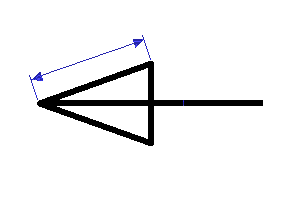Dimension and Annotation Leader Symbols: Transparent arrow angle (degrees)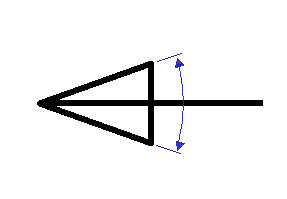Dimension and Annotation Leader Symbols: Slash size (mm)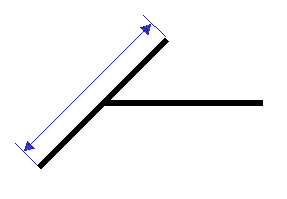Dimension and Annotation Leader Symbols: Outlined circle size (mm)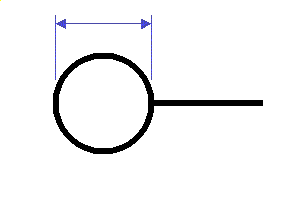Dimension and Annotation Leader Symbols: Filled circle size (mm)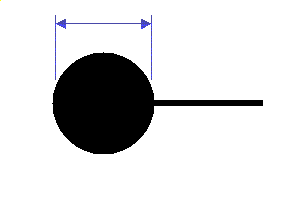Dimension and Annotation Leader Symbols: Transparent circle size (mm)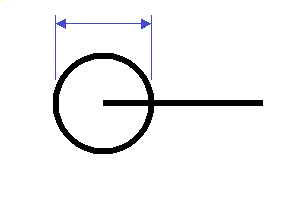Dimension and Annotation Leader Symbols: Crossed circle size (mm)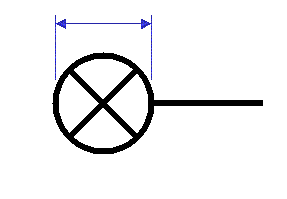Dimension and Annotation Leader Symbols: Outlined triangle size (mm)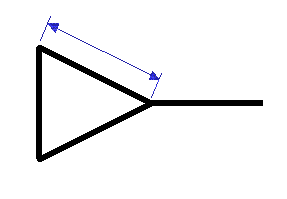Dimension and Annotation Leader Symbols: Filled triangle size (mm)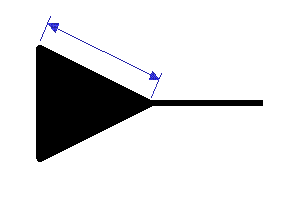Dimension and Annotation Leader Symbols: Plus size (mm)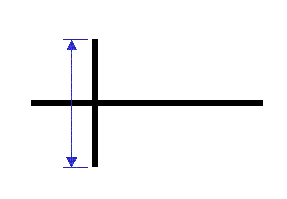Dimension and Annotation Leader Symbols: Cross size (mm)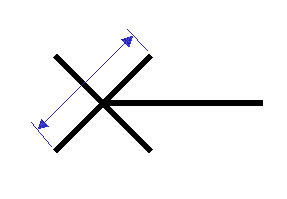Dimension and Annotation Leader Symbols: Symbol reversal limit (mm)

### Dimension Value

 Parameter Value Description Dimension Value: Underlining size, Left tail (mm)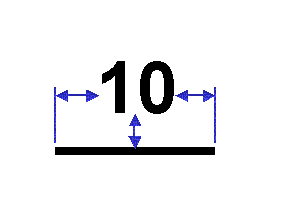Dimension Value: Underlining size, Right tail Dimension Value: Underlining size, Vertical space

### Chamfer Dimension Parameters

 Parameter Value Description Chamfer Dimension: Separator font height (mm)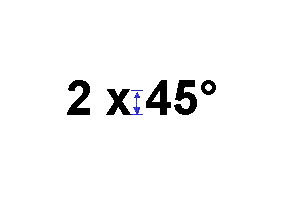Chamfer Dimension: Value framing [ 1 / 2 ] 1 = separately 2 = as a whole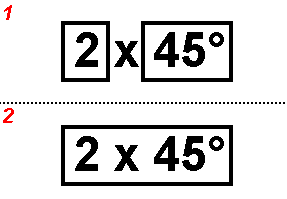### Half-Dimensions

 Parameter Value Description Half-Dimension: Dimension line extent (with value inside), Overrun mode [ 1 / 2 / 3 ] 1 = till Axis 2 = under value 3 = over axis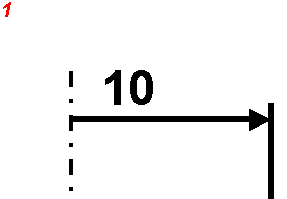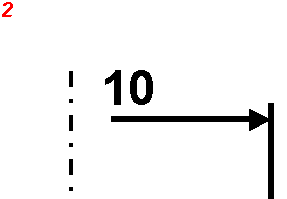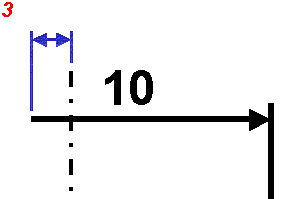Half-Dimension: Dimension line extent (with value inside), Overrun length (mm) if Half-Dimension: Dimension line extent (with value inside), Overrun mode = 3

### Dimension Associated Texts

 Parameter Value Description Dimension Associated Texts: Reference for positioning Before text 7 = top 8 = center 9 = bottom reference for positioning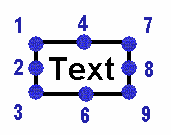for Before/After/Insert texts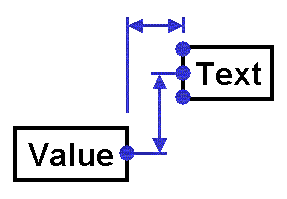for Upper/Lower texts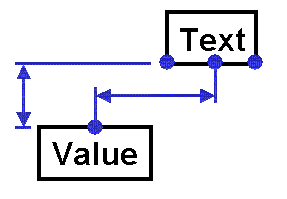Dimension Associated Texts: Reference for positioning After text 1 = top 2 = center 3 = bottom Dimension Associated Texts: Reference for positioning Insert text 1 = top 2 = center 3 = bottom Dimension Associated Texts: Reference for positioning Upper text 3 = left 6 = center 9 = right Dimension Associated Texts: Reference for positioning Lower text 1 = left 4 = center 7 = right Dimension Associated Texts: Horizontal offset of Before text (mm) Dimension Associated Texts: Vertical offset of Before text Dimension Associated Texts: Horizontal offset of After text Dimension Associated Texts: Vertical offset of After text Dimension Associated Texts: Horizontal offset of Insert text Dimension Associated Texts: Vertical offset of Insert text Dimension Associated Texts: Horizontal offset of Upper text Dimension Associated Texts: Vertical offset of Upper text Dimension Associated Texts: Horizontal offset of Lower text Dimension Associated Texts: Vertical offset of Lower text

### Annotations

 Parameter Value Description Annotation: Text angle [ No / Yes ] No = 0 to 360 degrees Yes = -90 to 90 degrees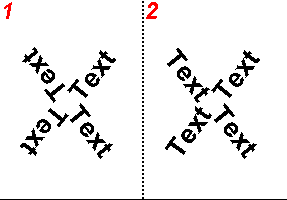Annotation: Text leader size, Leader side (mm) Warning: this parameter is used only for roughness symbols created before V5R12.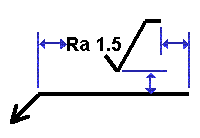Annotation: Text leader size, Opposite leader side (mm) Annotation: Text leader size, Vertical space (mm) Annotation: Text leader size, Leader gap (mm)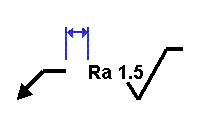Annotation: Text thickness (mm) For compatibility with V4. For dimensions created before R14 and annotations created before R13 SP4: non-bold fonts take this parameter into account. bold fonts are assigned a thickness of 0,7 mm (to preserve the previous behavior). Complex text and roughness annotations do not take this parameter into account. Deprecated for dimensions created since R14 and annotations created since R13 SP4. For these annotations and dimensions: bold fonts are assigned thickness index 3. non-bold fonts are assigned thickness index 1. Annotation: Datum feature leader representation mode [ 1 / 2 ] 1 = Normal 2 = Flag ANSI parent standard only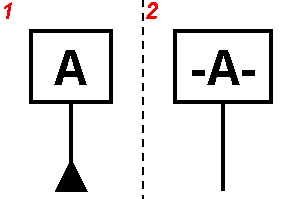### Fake dimensions

 Parameter Value Description Fake Dimension: Underline, Tail length (mm) If Fake Dimension: Value display mode = 1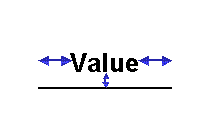Fake Dimension: Underline, Vertical offset

### Dual Dimensions

 Parameter Value Description Dual Dimension: Side-by-side dual display mode, Separator height (mm)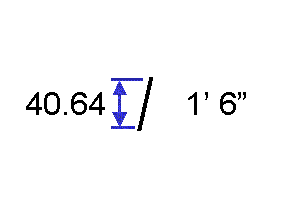Dual Dimension: Values above-one-another display mode, Above offset (mm)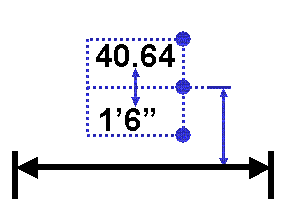Dual Dimension: Values above-one-another display mode, Above space (mm) Dual Dimension: Values above-one-another display mode, Position reference [1 / 2 / 3] 1 = top 2 = center 3 = bottom Dual Dimension: Values above-one-another display mode, Justification [1 / 2 / 3] 1 = left 2 = center 3 = right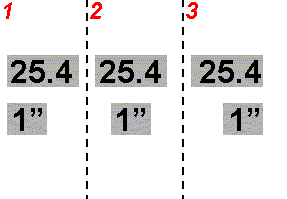### Table 1

Parameter

Value

Description

Cumulate Dimension: Sign display

[ 1 / 3 ]

1 = no sign
3 = positive sign on all values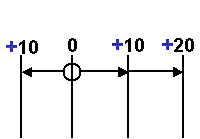Cumulate Dimension: Origin symbol shape

[ 0 / ... / 13 ]

0 = none
1-13 = refer to the dimension line symbols table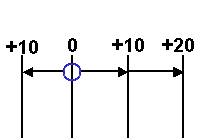Cumulate Dimension: Origin symbol scale

(real)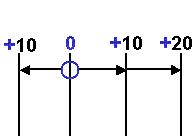Cumulate Dimension: Origin Extension Line Display

[ Yes  / No ]

Yes = display
No = no display

Note that only the first external line is modified.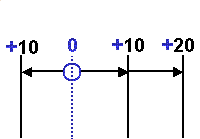Cumulate Dimension: Display of origin zero

[ Yes  / No ]

Yes = display
No = no display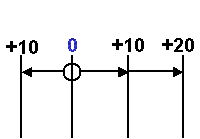[ 1 / 2 ]

1 = dimension line
2 = extension line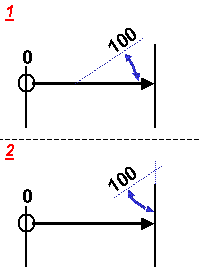Cumulate Dimension: Value orientation

[ 1 / 2 / 3 ]

1 = Parallel to Reference (specified by Cumulate Dimension: Value orientation reference)

2 = Perpendicular to Reference (specified by Cumulate Dimension: Value orientation reference)

3 = Angle to reference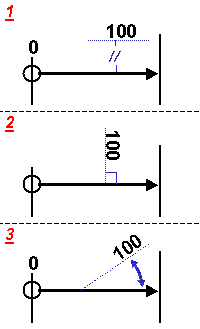Cumulate Dimension: Value orientation angle

(degrees)

if Cumulate Dimension: Value orientation=3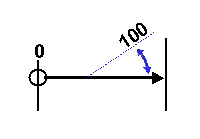### Table 2

Parameter

Value

Description

Cumulate Dimension: Dimension line length mode

[  2 / 3 / 4  ]

2 = Dimension Line to origin
3 = Length is relative to value text

4 = Length is constant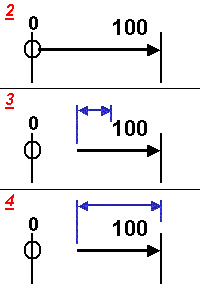Cumulate Dimension: Value vertical positioning, Justification

[ 1 / 2 ]

1 = Edge

2 = Center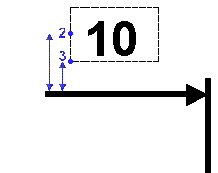CUMLTxtDecalY

-

Deprecated

Now managed by the Value > OffsetY parameter available for each Dimension Style.

If Dimension Line goes to origin
(Cumulate Dimension: Dimension line length mode = 2):

Cumulate Dimension: Value horizontal positioning, Justification

[ 1 / 2 ]

1 = Edge

2 = Center

For dimensions created before R14: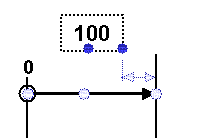For dimensions created in R14 and R15: see the note below.

For dimensions created from R16 onwards: this parameter is used if the dimension values alignment mode for cumulated dimension systems is defined as "From standard" in Dimension System Styles.

Cumulate Dimension: Value positioning reference

[ 1 / 2 / 3 ]

1 = Extension line

2 = Dim line center

3 = Origin

For dimensions created before R14: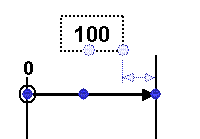For dimensions created in R14 and R15: see the note below.

For dimensions created from R16 onwards: this parameter is used if the dimension values alignment mode for cumulated dimension systems is defined as "From standard" in Dimension System Styles.

Cumulate Dimension: Value H/V positioning, Offset from orientation reference

(mm)

For dimensions created before R14: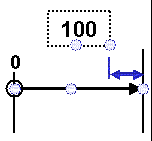For dimensions created in R14 and R15: see the note below.

For dimensions created from R16 onwards: this parameter is used if the dimension values alignment mode for cumulated dimension systems is defined as "From standard" in Dimension System Styles.

If Dimension Line is relative to value
(Cumulate Dimension: Dimension line length mode = 3):

Cumulate Dimension: Value horizontal positioning, Justification

[ 1 / 2 ]

1 = Edge

2 = Center

For dimensions created before R14: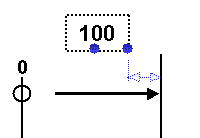For dimensions created in R14 and R15: see the note below.

For dimensions created from R16 onwards: this parameter is used if the dimension values alignment mode for cumulated dimension systems is defined as "From standard" in Dimension System Styles.

Cumulate Dimension: Value H/V positioning, Offset from orientation reference

(mm)

For dimensions created before R14: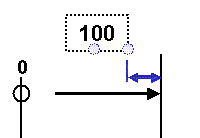For dimensions created in R14 and R15: see the note below.

For dimensions created from R16 onwards: this parameter is used if the dimension values alignment mode for cumulated dimension systems is defined as "From standard" in Dimension System Styles.

If Dimension Line has a constant length
(Cumulate Dimension: Dimension line representation = 4):

Cumulate Dimension: Dimension/Extension line length

(mm)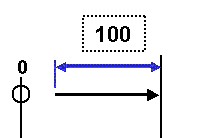Cumulate Dimension: Value horizontal positioning, Justification

[ 1 / 2 ]

1 = Edge

2 = Center

For dimensions created before R14: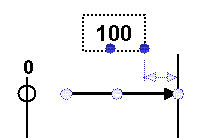For dimensions created in R14 and R15: see the note below.

For dimensions created from R16 onwards: this parameter is used if the dimension values alignment mode for cumulated dimension systems is defined as "From standard" in Dimension System Styles.

Cumulate Dimension: Value positioning reference

[ 1 / 2 / 3 ]

1 = Extension line

2 = Dim line center

3 = Origin

For dimensions created before R14: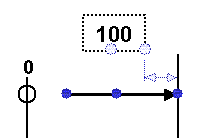For dimensions created in R14 and R15: see the note below.

For dimensions created from R16 onwards: this parameter is used if the dimension values alignment mode for cumulated dimension systems is defined as "From standard" in Dimension System Styles.

Cumulate Dimension: Value H/V positioning, Offset from orientation reference

(mm)

For dimensions created before R14: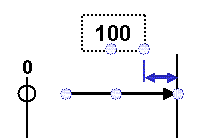For dimensions created in R14 and R15: see the note below.

For dimensions created from R16 onwards: this parameter is used if the dimension values alignment mode for cumulated dimension systems is defined as "From standard" in Dimension System Styles.A number of parameters applying only if value orientation reference is "Dimension Line" were deprecated for dimensions created in R14 and R15. In this case, cumulate dimensions were created within dimension systems and if the dimension value orientation reference was the dimension line, then the value horizontal positioning was defined by the following Dimension System Styles: Aligned cumulated dimension values and Values Offset.

### Table 3

Parameter

Value

Description

Cumulate Dimension: Dimension line representation

[ 1 / 2 / 4 ]

1 = no display
2 = full display
4 = partial length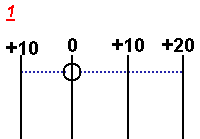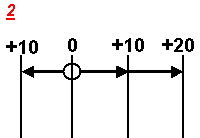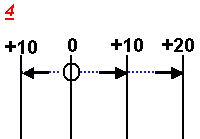Cumulate Dimension: Dimension line length

(mm)

if Dimension Line has a partial length
(Cumulate Dimension: Dimension line representation=4)Cumulate Dimension: Extension line length mode

[ 3 / 4 ]

3 = relative to text box
4 = constant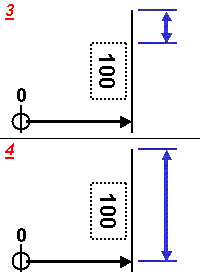If extension line is relative to value text (Cumulate Dimension: Extension line length mode = 3):

Cumulate Dimension: Extension line overrun

(mm)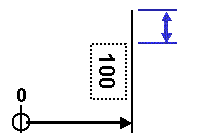Cumulate Dimension: Value vertical positioning, Justification 2

[ 1 / 2 ]

1 = Edge

2 = Center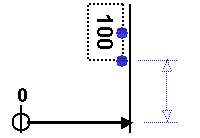Cumulate Dimension: Value HV positioning, offset from orientation reference

(mm)

Deprecated

Now managed by the Value > OffsetX parameter available for each Dimension Style

Cumulate Dimension: Value horizontal positioning, Justification

[ 1 / 2 ]

1 = Edge

2 = Center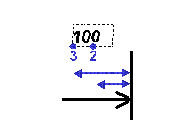CUMLExtLTxtHPos

-

Deprecated

Now managed by the Value > OffsetY parameter available for each Dimension Style

If extension line is constant (Cumulate Dimension: Extension line length mode = 4):

Cumulate Dimension: Dimension/Extension line length

(mm)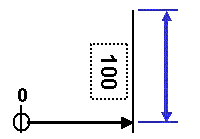Cumulate Dimension: Value vertical positioning, Justification 2

[ 1 / 2 ]

1 = Edge

2 = Center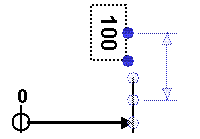Cumulate Dimension: Value positioning reference

[ 1 / 2 / 3 ]

1 = Dimension line

2 = Middle of extension line

3 = Extension line end point (opposite to dimension line)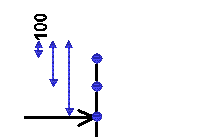Cumulate Dimension: Value HV positioning, offset from orientation reference

(mm)

Deprecated

Now managed by the Value > OffsetX parameter available for each Dimension Style.

Cumulate Dimension: Value horizontal positioning, Justification

[ 1 / 2 ]

1 = Edge

2 = CenterCUMLExtLTxtHPos

(mm)

Deprecated

Now managed by the Value > OffsetY parameter available for each Dimension Style.

### Curvilinear Length Symbol

 Option Description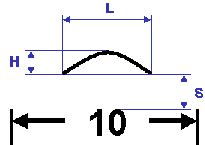Display Symbol Specifies whether the curvilinear length symbol should be displayed. Height Indicates the height (in mm) of the curvilinear length symbol. Spacing Indicates the spacing (in mm) between the curvilinear length symbol and the dimension value. Underline value Specifies whether the dimension value should be underlined. Length Indicates the length (in mm) of the curvilinear length symbol. Minimum Length Indicates the minimum length (in mm) of the curvilinear length symbol. Minimum Length Indicates the maximum length (in mm) of the curvilinear length symbol.

### Intersection Point

 Option Description Print intersection points Specifies whether the intersection point should be printed. If you leave this option unchecked, then the intersection point will be a construction point and its style will be the default construction point style as defined in the Styles > Point > Default section of the standard. If you check this option, then the intersection point will not be a construction point and its style can be chosen among the various point styles defined in the Styles > Point section of the standard. Point style Indicates the style that should be used to represent the point (as defined in the Styles > Point section of the standard). Show construction lines Specifies whether construction lines should be displayed. Print construction lines Specifies whether construction lines should be printed. This option is available when the Show construction lines option is checked. Line style Specifies the style that should be used to represent the construction line (as defined in the LineTypes section of the standard).